﻿ Generate Random Numbers using R Script on SQL ServerSQL Server and T-SQL Development Tutorials Development resources, articles, tutorials, code samples, tools and downloads for ASP.Net, SQL Server, Reporting Services, T-SQL, Windows, AWS, SAP HANA and ABAP

# Generate Random Numbers using R Script on SQL Server

For database developers it is possible to use R script on SQL Server to generate random numbers. With SQL Server 2016 and SQL Server 2017 Machine Learning Services (either In-Database or as Standalone), data engineers are able to run R scripts and Python (new in SQL Server 2017) within T-SQL codes.
R services and Python provides powerful libraries for especially for data analytics. In this R in SQL tutorial, I will share how to create random numbers or generate random integer on SQL Server using R-services.

If you are familiar with executing R scripts on SQL Server, for random number generation you can refer to Uniform Distribution functions, random articles for details.

Following R Script generates a random number between 0 and 1.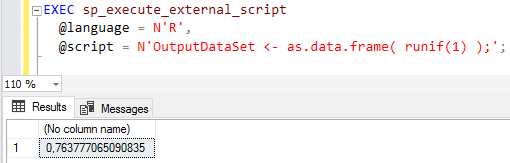In fact, RUNIF function takes 3 arguments. In above R code we have only used one parameter which is equal to 1.
This input argument enables RUNIF function to create that amount of numbers to be generated as output.

For example, if you modify above R in SQL script as follows and execute it, you will have 3 random numbers generated between 0 and 1.Other parameters are minimum and maximum values defines the range boundaries where the random number will be created within.
If SQL developer executes following sample code, 4 random numbers will be generated between 0 and 10.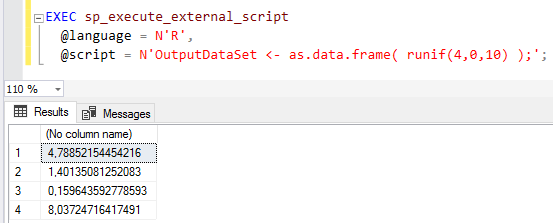Below R script executed using sp_execute_external_script in SQL generates a random number and returns this randomly created number using an OUTPUT parameter.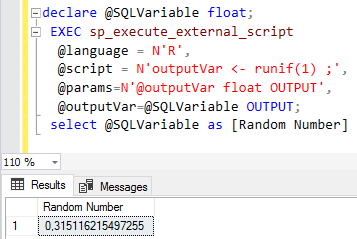Below code generates a random integer between desired two integers. For example, it is possible to create random number between 0 and 100 by setting variable values in below T-SQL code.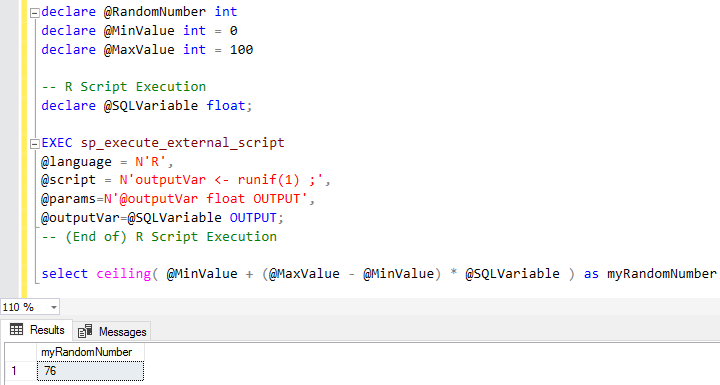Another method of creating any number of random integers between a desired number range is as follows: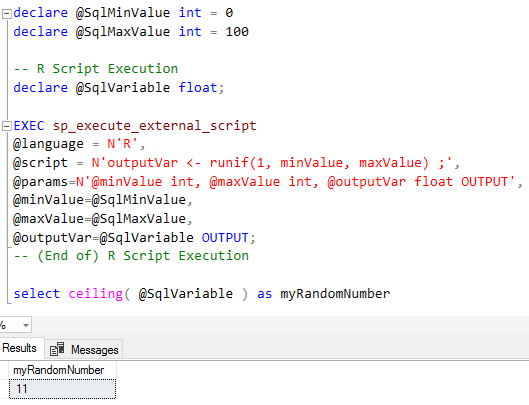As SQL Server data engineers can realize, I used input parameters and output parameters to pass and read data from SQL stored procedure sp_execute_external_script to execute parametric R script

I encapsulated previous SQL codes and R script which generate random number in a SQL stored procedure.

Here it is an example for SQL Server database developers showing how to call and execute SQL stored procedure to create random numberSQL Server